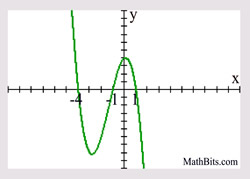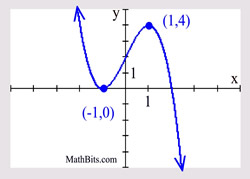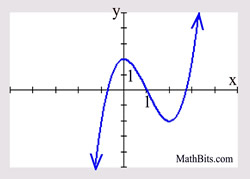Graphing Factorable Polynomials Terms of Use    Contact Person: Donna Roberts1.
Given: P(x) = x2 + 2x - 24
At what x-values will the graph cross the x-axis?Choose:
 x = +6, x = -4 x = +3, x = -8 x = +4, x = -6 x = -3, x = 8

2.
A polynomial function is defined to be
f (x) = (x + 3)(x - 2)2.
Which choice describes the graph's behavior at the x-axis?Choose:
 The graph only touches the x-axis at x = -3, but crosses the x-axis at x = 2. The graph only touches the x-axis at x = 3, but crosses the x-axis at x = -2. The graph only touches the x-axis at x = 2, but crosses the x-axis at x = -3. The graph only touches the x-axis at x = -2, but crosses the x-axis at x = 3.

3.
Given:  the graph at the right.
Which choice lists possible factors of this polynomial function?Choose:
 (x - 4)(x - 1)(x + 1) x = -4; x = -1; x = 1 x = 4; x = 1; x = -1 (x + 4)(x + 1)(x - 1)

4.
Given: the graph at the right.
Which choice is the y-value of the relative maximum reached by this graph?Choose:
 1 0 none (approaches +∞) 4

5.
Given: the graph at the right.
Which choice best describes the polynomial function associated with this graph based upon the graphs "end behaviors"?

Choose:the degree is even and the leading coefficient is negative the degree is even and the leading coefficient is positive the degree is odd and the leading coefficient is negative the degree is odd and the leading coefficient is positive

6.
Given: P(x) = x3 + 6x2 + 8x
Which choice shows the roots of this polynomial function?
Choose:
 x = 0, -2, -4 x = 0, 2, -4 x = 0, 2, 4 x = 0, -2, 47.
Given: P(x) = x2 - 2x - 48
Which choice shows the x-intercepts of this polynomial function?Choose:
 (-8,0), (6,0) (-6,0), (8,0) (0,-8), (0,6) (0,-6), (0,8)

8.
Given: P(x) = x2 - 6x + 9
Which choice states the number of x-intercepts of this polynomial function?Choose:
 1 2 3 0